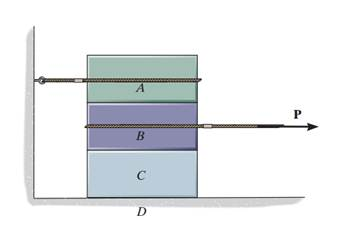### Determine the smallest horizontal force p

Assignment Help Basic Computer Science
##### Reference no: EM131035157

Blocks A, B, and C have weights of 50 lb, 25 lb, and 15 lb, respectively. Determine the smallest horizontal force P that will cause impending motion. The coefficient of static friction between A and B is µ's = 0.3 , between B and C, µ's = 0.4 and between block C and the ground, m's = 0.35#### Programming looping structures

Looping structures are key design structures used in programming languages. The ability to repeat code is a powerful tool and can save many lines of code; however, if a loop

#### Levels of confidence and convenience

Explain how levels of confidence and convenience (however small or large they are) comparable to the ones in the question of Third Protocol Homework Assignment can be achiev

#### Determine whether r is reflexive-irreflexive-symmetric

A whose matrix is attached below. Determine whether R is reflexive, irreflexive, symmetric, asymmetric, antisymmetric, or transitive. For each property, either explain why R

#### Define a polygon adt to represent a geometric polygon

A polygon is a closed geometric shape consisting of three or more line segments that are connected end to end. The endpoints of the line segments are known as vertices, whic

#### Determine the energy of violet light

If you were required to write a computer program to determine the energy of a photon of different light wavelengths (such as red, green, and so forth), what inputs, outputs,

#### Risk-disasters handling plan

Many companies have a "risk action plan" to handle unexpected events including network security event. Tutor, is your company or a company which you know has risk/disasters

#### What are the differences between ospf and is is

For this Discussion Board, you need to really understand what a link-state protocol is. In a previous assignment, you were asked to describe them. In your own words, answer

#### Distance between nodes u and v

The distance between nodes u and v in graph is the length of the shortest path from u to v. Given a d-dimensional hypercube and a designated source node s, how many nodes are

### Write a Review